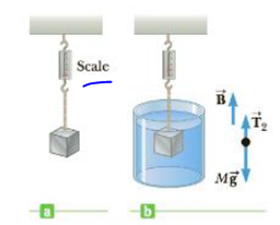Chapter 9, Problem 26P

Chapter
Section
Textbook Problem

The gravitational force exerted on a solid object is 5.00 N as measured when the object is suspended from a spring scale as in Figure P9.26a. When the suspended object is submerged in water, the scale reads 3.50 N (Fig. P9.26b). Find the density of the object.Figure P9.26

To determine
The density of the object.

Explanation
The sum of forces on the object after it submerged in water would be written as Fy=0=B+Fg,apparantFg,actual and the force of buoyant is B=ρwatergVobject and the volume of the object would be rearranged from the buoyant force as Vobject=B/(ρwaterg) . This expression of volume is used in general definition of the density of an object as follows ρobject=mobject/Vobject and mobjectg=Fg,actual

Given info: Weight or actual weight of the object is 5.00N , density of water is 103kg/m3 , acceleration due to gravity is 9.80m/s2 , and apparent weight of the object is 3.50N .

The formula for density of the object is,

ρobject=(Fg,actualFg,actualFg,apparant)ρwater

• Fg,actual is actual weight of the object

Still sussing out bartleby?

Check out a sample textbook solution.

See a sample solution

The Solution to Your Study Problems

Bartleby provides explanations to thousands of textbook problems written by our experts, many with advanced degrees!

Get Started

Find more solutions based on key concepts